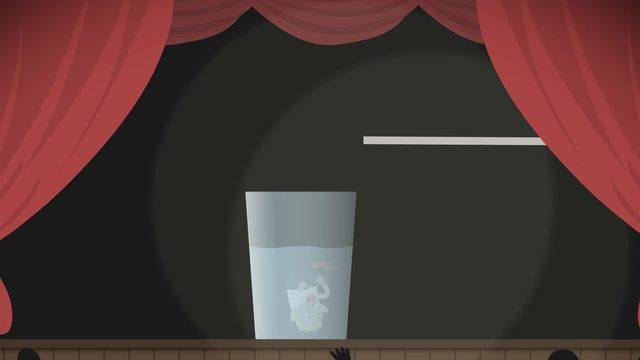# Using a Ruler, Protractor, and CompassRating

Ø 4.2 / 42 ratings
The authorsChris S.

## Basics on the topicUsing a Ruler, Protractor, and Compass

After this lesson, you will be able to use a ruler and protractor to measure segments and angles, and a compass to copy segments without measuring.

The lesson begins by teaching you to measure and sketch segments with a ruler. Then you will learn to measure and sketch angles with a protractor. It concludes with creating exact copies of segments with a compass.

Learn about the essential tools of geometry by helping Harry and Lloyd sell their wares!

This video includes key concepts and vocabulary such as ruler (a tool for measuring segments and drawing segments of specific length); protractor (a tool for measuring angles and drawing angles of specific measure); and compass (a tool for creating circles and copying segments, all without measuring).

Before watching this video, you should already be familiar with segments, lines, rays, angles, units of length, degree measures, and a variety of geometric shapes.

After watching this video, you will be prepared to learn how to use a set square to construct a parallelogram, and how to use a compass to construct a triangle.

Common Core Standard(s) in focus: 7.G.A.2 A video intended for math students in the 7th grade Recommended for students who are 12 - 13 years old

### TranscriptUsing a Ruler, Protractor, and Compass

Harry and Lloyd have been inching across the country at a snail's pace, collecting whatever they can find and trying to offload it on unsuspecting customers. They've recently found some strange geometric tools on their journey, but they don't know how they work. Let's see if we can help Harry and Lloyd find out how to use a ruler, protractor, and compass. Here's the first item: a ruler! Woah! Kids, don't try this at home! So what's a ruler really good for? First off, we can use it as a straight-edge, to construct segments rays and lines. Second, we can use a ruler to measure lengths. The tick marks help us discover how long a line segment is in inches or in centimeters. How long is this segment? It is 5 inches long. It's also 12.7 centimeters. Finally, we can draw segments of a desired length. If we want a four inch segment we start here and end here. When a measurement is between the tick marks, we can't be completely sure how long it is. That's why measurements with rulers are always approximations. Now, what is this thing for? Right. Of course! Actually, this is a protractor. We use it to measure angles and construct angles. A protractor is marked with the 180 degrees of a semi-circle. The center of the circle is here. Zero degrees is here and 180 degrees is here. But 0 to 180 is marked in reverse too. Why do they have the angle markings listed twice on a protractor? So we can measure angles that start on either the left side or the right side. To measure an angle place the center of the protractor at the vertex of the angle and match up the baseline of the protractor with one side. Find where the other side crosses the protractor and read the degrees there. Which of the two numbers is the measure of our angle? We need to make sure to choose from the same row that shows 0 degrees for the initial side. 130 degrees! We can also use a protractor to draw an angle of specific measure. Let's draw a 40 degree angle. First, we draw a vertex and an initial side. Then, place the center of the protractor on the vertex and line up the baseline. Where do we draw the terminal side of the angle? Look for 40 degrees in the correct row put a point there then draw the terminal side of the angle. Done and "done-er"! Back to Harry and Lloyd. What's this contraption for? This is a compass. It consists of two legs connected at a hinge. One leg has a point at the end while the other has a pencil. We can adjust the distance between the point and the pencil and when it's fixed, it should stay at that distance. With a compass we can construct circles, as well as copy segments without measuring. To construct a circle, create a center point put the needle there and rotate the compass. The distance between the needle and the pencil stays the same, so we know we have a circle. Now let's make a copy of this line segment. First, choose where one of the endpoints of the copy will be. Next, we match the needle and pencil with the endpoints of the given segment. The length of the line segment is now saved in the compass as the distance from the needle to the pencil. Now, we make an ARC from the new endpoint. Any segment from here to the arc will be a perfect copy. For example, this line segment. Using a compass is better than using a ruler since it gives us an exact copy, and the ruler only gave us an approximate value for the length. That's why it's an important tool for geometric proofs. Before we see what Harry and Lloyd did with their compass, let's review the geometric tools we explored. A ruler is for measuring segments and drawing segments of a specific length. A protractor can be used to measure angles as well as draw angles of a specific measure. A compass constructs circles and copies segments without measuring. Let's see if Harry and Lloyd have discovered how to use these geometric tools properly. Guess not.

1 comment
1 comment
1.It’s okay

From Brayden, about 1 year ago